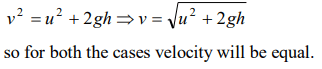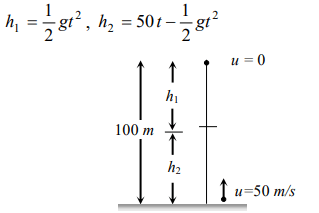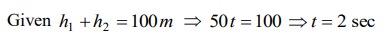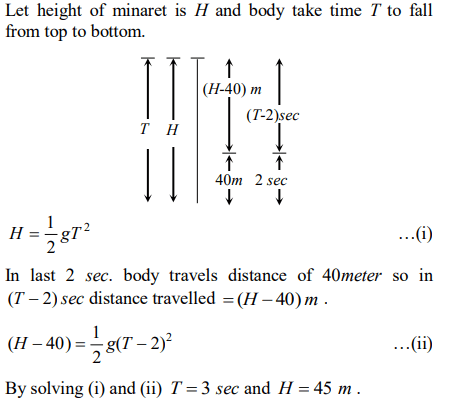## Motion in a Straight Line Questions and Answers Part-18

1. A man throws a ball vertically upward and it rises through 20 m and returns to his hands. What was the initial velocity $\left(u\right)$ of the ball and for how much time $\left(T\right)$ it remained in the air$\left(g=10 m\diagup s^{2}\right)$
a) u = 10 m/s, T = 2s
b) u = 10 m/s, T = 4s
c) u = 20 m/s, T = 2s
d) u = 20 m/s, T = 4s

Explanation:2. A particle when thrown, moves such that it passes from same height at 2 and 10s, the height is
a) g
b) 2 g
c) 5 g
d) 10 g

Explanation:3. Three different objects of masses $m_{1},m_{2}$   and  $m_{3}$  are allowed to fall from rest and from the same point ‘O’ along three different frictionless paths. The speeds of the three objects, on reaching the ground, will be in the ratio of
a) $m_{1}:m_{2} :m_{3}$
b) $m_{1}:2m_{2} :3m_{3}$
c) $1:1: 1$
d) $\frac{1}{m_{1}}:\frac{1}{m_{2}}:\frac{1}{m_{3}}$

Explanation:4. From the top of a tower, a particle is thrown vertically downwards with a velocity of 10 m/s. The ratio of the distances, covered by it in the 3$^{rd}$ and $2^{nd}$ seconds of the motion is $\left(g=10 m\diagup s^{2}\right)$
a) $5:7$
b) $7:5$
c) $3:6$
d) $6:3$

Explanation:5. Two balls A and B of same masses are thrown from the top of the building. A, thrown upward with velocity V and B, thrown downward with velocity V, then
a) Velocity of A is more than B at the ground
b) Velocity of B is more than A at the ground
c) Both A & B strike the ground with same velocity
d) None of these

Explanation:6. A ball is dropped from top of a tower of 100m height. Simultaneously another ball was thrown upward from bottom of the tower with a speed of 50 m/s$\left(g=10 m\diagup s^{2}\right)$    . They will cross each other after
a) 1s
b) 2s
c) 3s
d) 4s

Explanation:7. A cricket ball is thrown up with a speed of $19.6 ms^{-1}$  . The maximum height it can reach is
a) 9.8 m
b) 19.6 m
c) 29.4 m
d) 39.2 m

Explanation:8. A very large number of balls are thrown vertically upwards in quick succession in such a way that the next ball is thrown when the previous one is at the maximum height. If the maximum height is 5m, the number of ball thrown per minute is $\left(g=10 m s^{-2}\right)$
a) 120
b) 80
c) 60
d) 40

Explanation:9. A body falling from a high Minaret travels 40 meters in the last 2 seconds of its fall to ground. Height of Minaret in meters is $\left( g=10 m\diagup s^{2}\right)$
a) 60
b) 45
c) 80
d) 50a) $1:4:9$
b) $1:2:4$
c) $1:3:5$
d) $1:2:3$